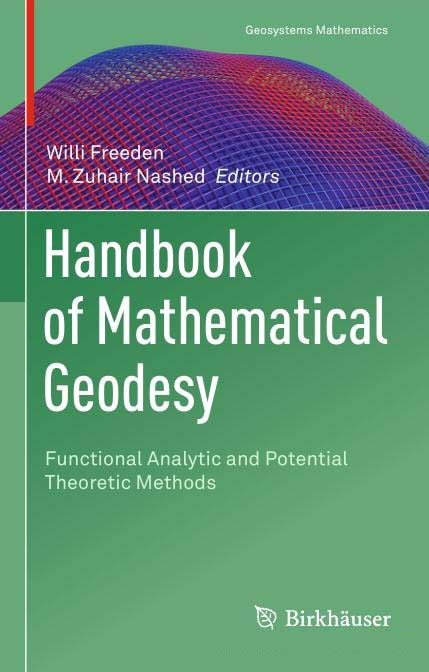# Handbook of Mathematical Geodesy: Functional Analytic and Potential Theoretic Methods

#### Category: Nonfiction

Posted on 2019-07-12, by winzham.

Description

Written by leading experts, this book provides a clear and comprehensive survey of the "status quo" of the interrelating process and cross-fertilization of structures and methods in mathematical geodesy. Starting with a foundation of functional analysis, potential theory, constructive approximation, special function theory, and inverse problems, readers are subsequently introduced to today's least squares approximation, spherical harmonics reflected spline and wavelet concepts, boundary value problems, Runge-Walsh framework, geodetic observables, geoidal modeling, ill-posed problems and regularizations, inverse gravimetry, and satellite gravity gradiometry. All chapters are self-contained and can be studied individually, making the book an ideal resource for both graduate students and active researchers who want to acquaint themselves with the mathematical aspects of modern geodesy.Handbook of Mathematical Geodesy

8282 dl's @ 2708 KB/s
5622 dl's @ 2159 KB/s
5864 dl's @ 2229 KB/s

Search More...
Handbook of Mathematical Geodesy: Functional Analytic and Potential Theoretic MethodsRelated Books